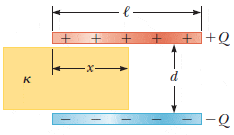# Inserting a dielectric between the plates of a parallel plate capacitor

• archaic
In summary, the conversation discussed a series of equations related to calculating electric charge, potential energy, and force. The equations involved variables such as capacitance, distance, and voltage. The conversation also mentioned a possible mistake in the calculated force, but it was determined to be correct.

#### archaic

Homework Statement
We have a capacitor made of two square plates of length ##\ell##, and separated by a distance ##d##. Charges ##+Q## and ##-Q## are placed on the plates.
A material of dielectric constant ##\kappa=4.50## is inserted a distance ##x## into the capacitor as shown in the figure below.
The system can be considered as two capacitors connected in parallel.
a) Find the equivalent capacitance.
b) Calculate the energy stored in the capacitor.
c) Find the direction and magnitude of the force exerted by the plates on the dielectric.
b) If the capacitor was charge to ##2.20\times10^3## V before the dielectric was inserted, then give a numerical value of the force in micro newtons if ##x=\ell/2##, ##\ell=5.10## cm, and ##d=1.80## mm.
Relevant Equations
$$C=\frac{Q}{\Delta V}$$a)$$C=\frac{\kappa\epsilon_0\ell x}{d}+\frac{\epsilon_0\ell(\ell-x)}{d}=\frac{\epsilon _0ℓ\left(\kappa x+ℓ-x\right)}{d}$$
b)$$U=\frac{1}{2C}Q^2=\frac{dQ^2}{2\epsilon _0ℓ\left(\kappa x+l-x\right)}$$
c)$$F=-\frac{dU}{dx}=\frac{dQ^2\left(\kappa -1\right)}{2\epsilon _0ℓ\left(\kappa x+ℓ-x\right)^2}$$and it is directed to the right, since it is positive.
b) I first find the charge using ##Q=C_0\Delta V=\frac{\epsilon_0\ell^2\Delta V}{d}##\begin{align*} F&=\frac{d\left(\kappa -1\right)}{2\epsilon _0ℓ\left(\kappa\fracℓ2+ℓ-\fracℓ2\right)^2}\frac{\epsilon_0^2\ell^4(\Delta V)^2}{d^2}\\ &=\frac{d\left(\kappa -1\right)}{2\epsilon _0\frac{ℓ^3}{4}\left(\kappa+1\right)^2}\frac{\epsilon_0^2\ell^4(\Delta V)^2}{d^2}\\ &=\frac{2\epsilon_0\ell(\Delta V)^2(\kappa-1)}{d(\kappa+1)^2}\\ &=\frac{2(5.10\times10^{-2})(2.20\times10^3)^2(4.50-1.00)}{(4\pi\times8.99\times10^9)(1.80\times10^{-3})(4.50+1)^2}\\ &=0.000281\,N=281\,\mu N \end{align*}

I think that I have a mistake in my force calculation, but I can't see it..

archaic said:
I think that I have a mistake in my force calculation, but I can't see it..
What makes you think it is wrong?

haruspex said:
What makes you think it is wrong?
I have found the same exercise in my textbook, but with different values, and when I plug their values I get a different answer.
It might be that they are wrong..
Do you see no mistakes?

archaic said:
I have found the same exercise in my textbook, but with different values, and when I plug their values I get a different answer.
It might be that they are wrong..
Do you see no mistakes?
I can't see any. Didn't check the numerical result precisely, but it is in the ballpark.

•archaic
haruspex said:
I can't see any. Didn't check the numerical result precisely, but it is in the ballpark.
It was the correct answer after all.. Thanks!